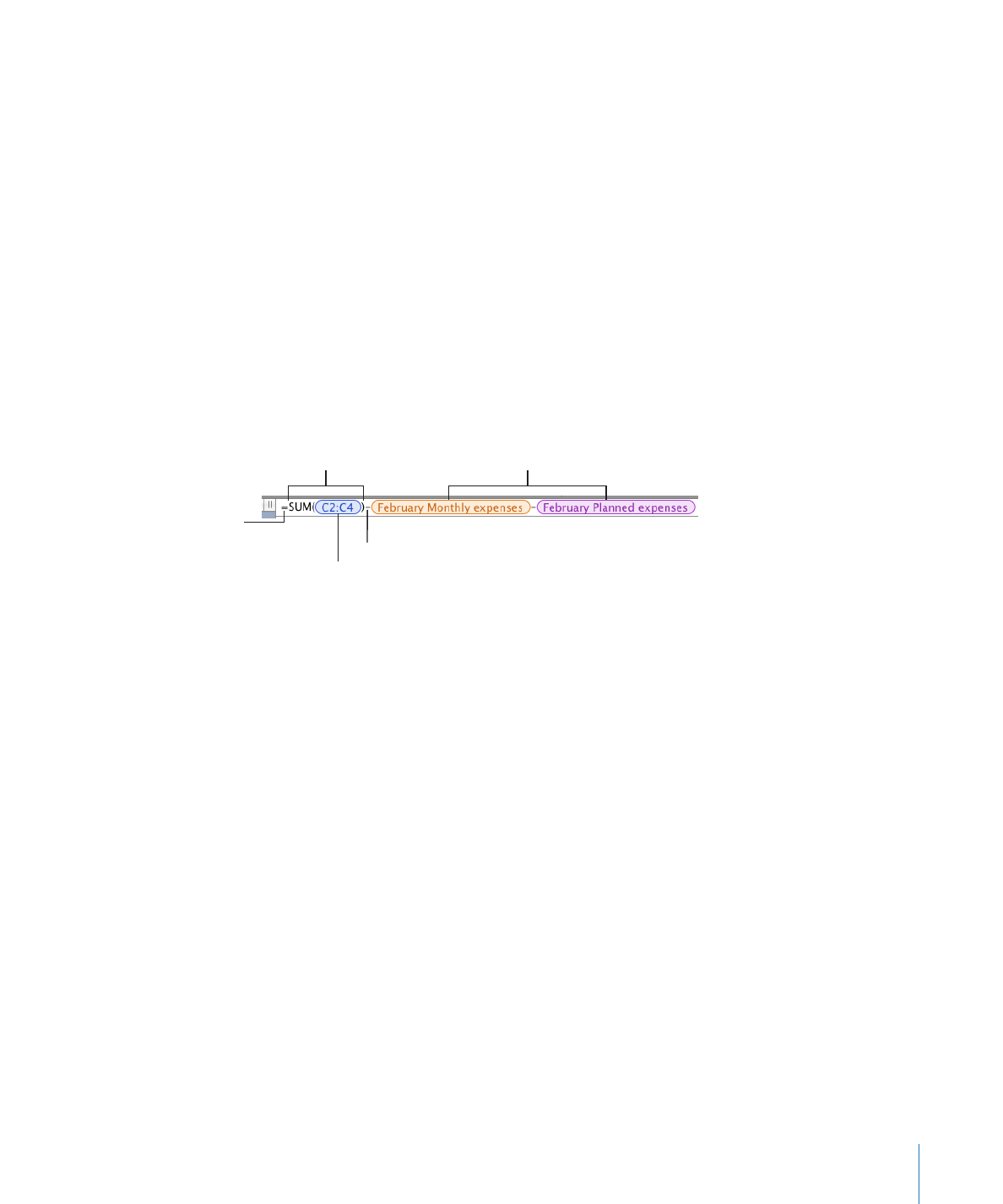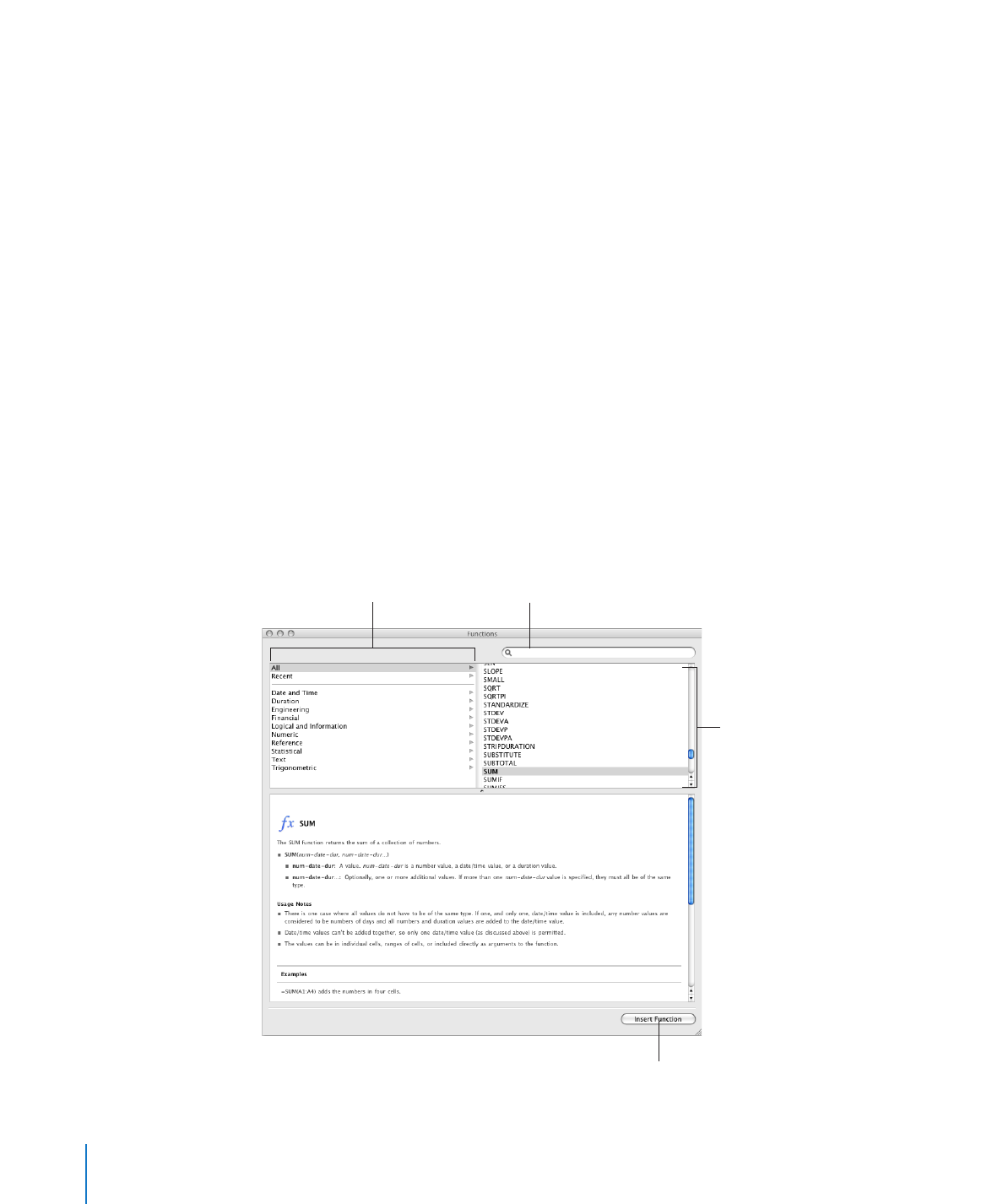## Numbers - Adding and Editing Formulas Using the Formula BarAdding and Editing Formulas Using the Formula Bar

The formula bar, located beneath the format bar, lets you create and modify formulas
in a selected cell. As you add cell references, operators, functions, or constants to a
formula, they appear like this.

The Subtraction operator.

References to cells
using their names.

The Sum function.

All formulas must begin

with the equal sign.

A reference to a
range of three cells.

Here are ways to work with the formula bar:
To add or edit a formula, select the cell and add or change formula elements in the

m

formula bar.

m

To add an operator or a constant, place the insertion point in the formula bar and

Â

type. You can use the arrow keys to move the insertion point around. See “Using
Operators in Formulas” on page 130 to learn about operators you can use. When
your formula requires an operator and you haven’t added one, the + operator is
inserted automatically. Select the + operator and type a different operator if needed.
To add cell references to the formula, place the insertion point and follow the

Â

instructions in “Referring to Cells in Formulas” on page 126.
To add functions to the formula, place the insertion point and follow the

Â

instructions in “Adding Functions to Formulas” on page 124.

To increase or decrease the display size of formula elements in the formula bar, choose

m

an option from the Formula Text Size pop-up menu above the formula bar.
To increase or decrease the height of the formula bar, drag the resize control at the
bottom right of the formula bar down or up, or double-click the resize control to auto-
fit the formula.To remove an element from the formula, select the element and press Delete.

m

To save changes, press Return, press Enter, or click the Accept button above the

m

formula bar. You can also click outside the formula bar.
To avoid saving any changes you made, click the Cancel button above the formula bar.